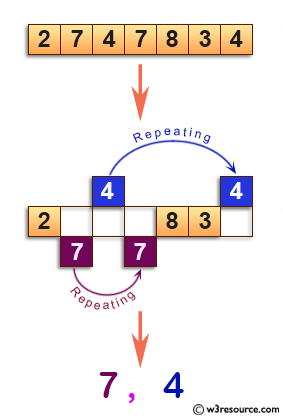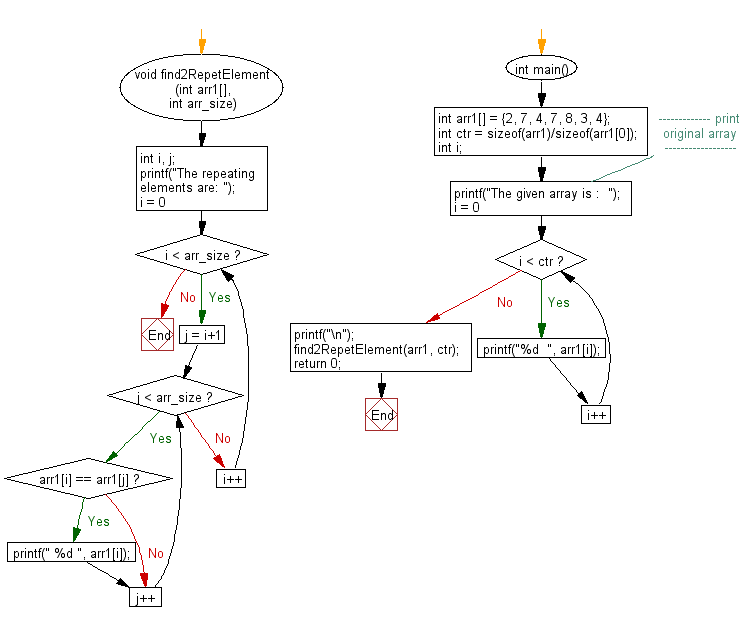﻿ C exercises: Find the two repeating elements in a given array - w3resource# C Exercises: Find the two repeating elements in a given array

## C Array: Exercise-44 with Solution

Write a program in C to find the two repeating elements in a given array.

Pictorial Presentation:Sample Solution:

C Code:

``````#include<stdio.h>
#include<stdlib.h>
void find2RepetElement (int arr1[], int arr_size)
{
int i, j;
printf("The repeating elements are: ");
for(i = 0; i < arr_size; i++)
for(j = i+1; j < arr_size; j++)
if(arr1[i] == arr1[j])
printf(" %d ", arr1[i]);
}

int main()
{
int arr1[] = {2, 7, 4, 7, 8, 3, 4};
int ctr = sizeof(arr1)/sizeof(arr1);
int i;
//------------- print original array ------------------
printf("The given array is :  ");
for(i = 0; i < ctr; i++)
{
printf("%d  ", arr1[i]);
}
printf("\n");

find2RepetElement(arr1, ctr);
return 0;
}
```
```

Sample Output:

```The given array is :  2  7  4  7  8  3  4
The repeating elements are:  7  4
```

Flowchart:C Programming Code Editor:

Improve this sample solution and post your code through Disqus.

What is the difficulty level of this exercise?

﻿